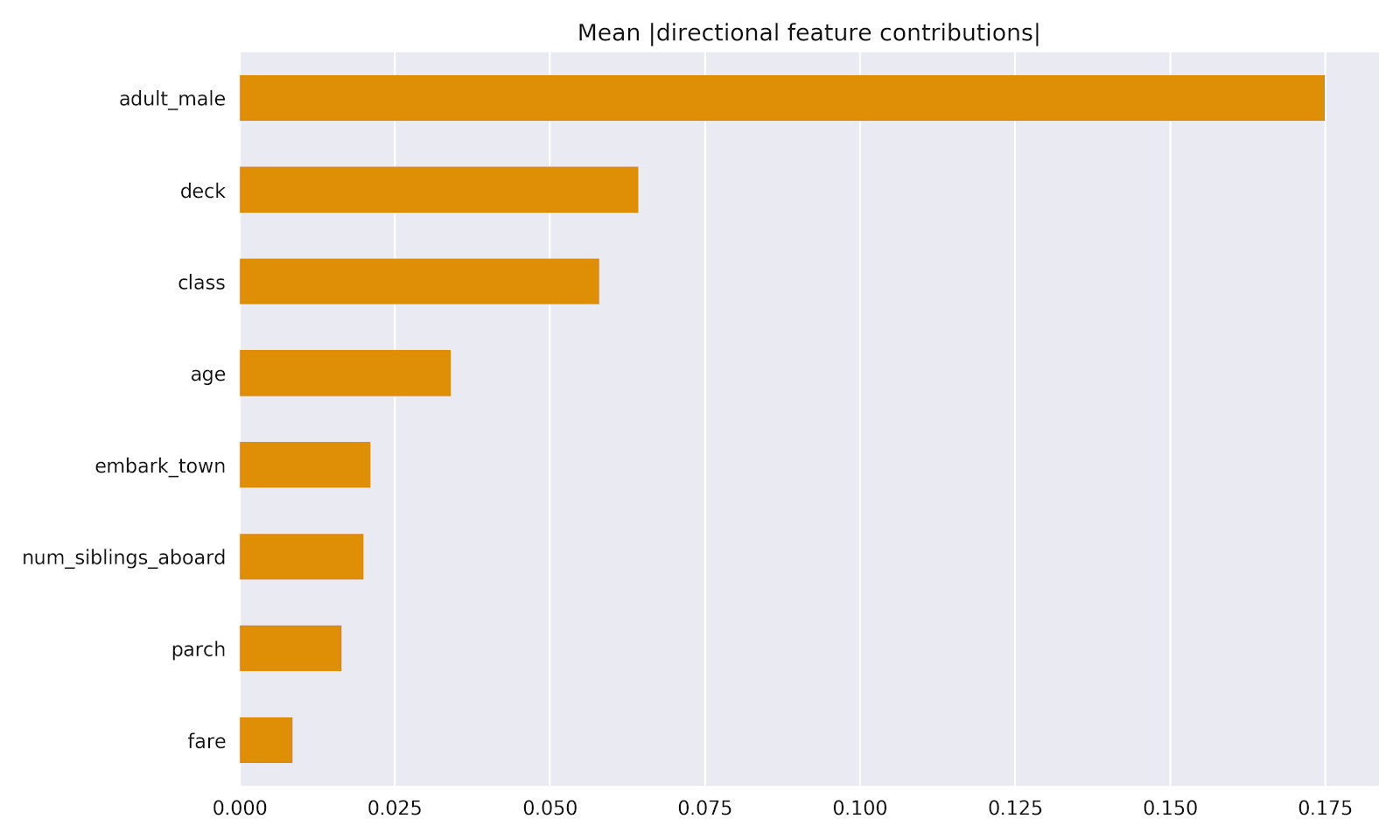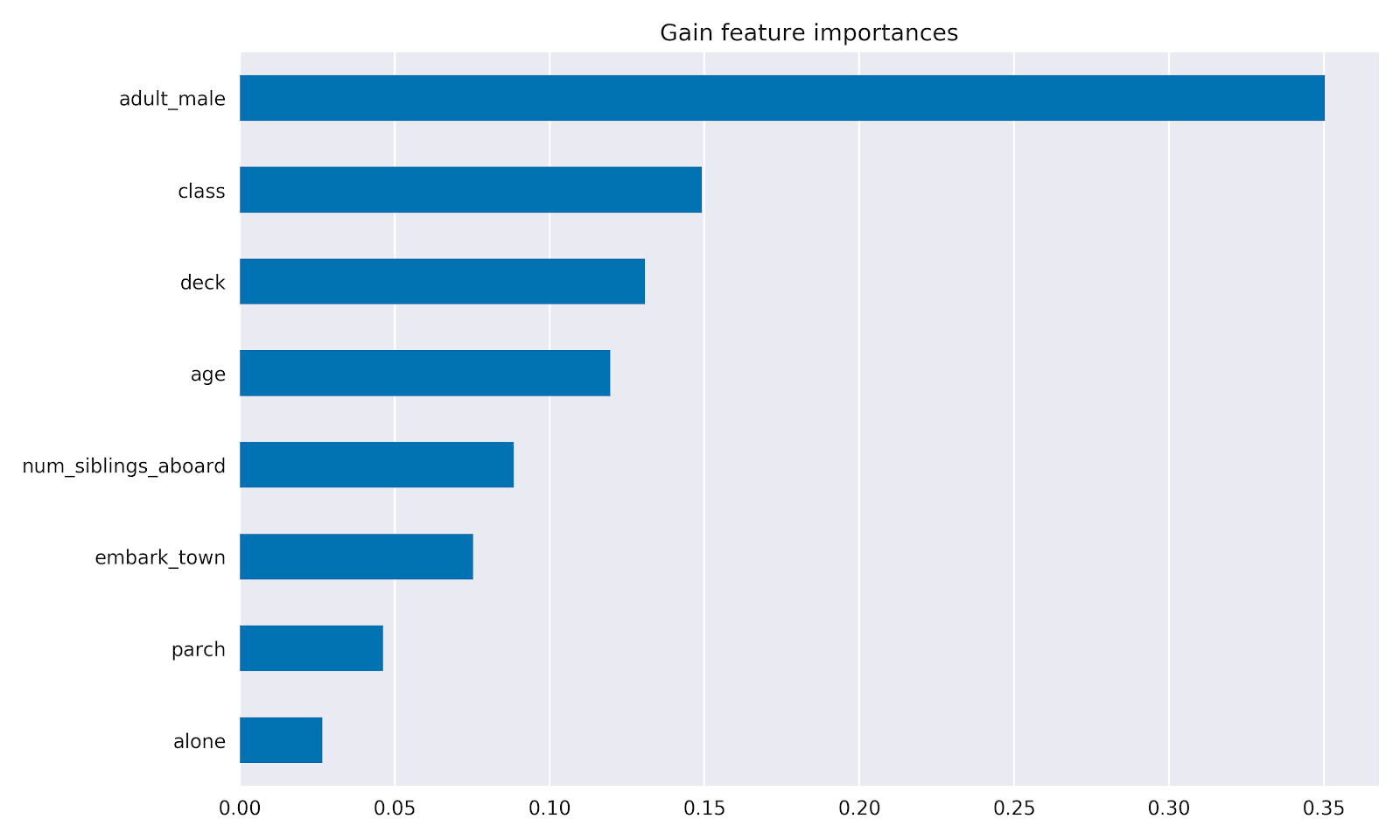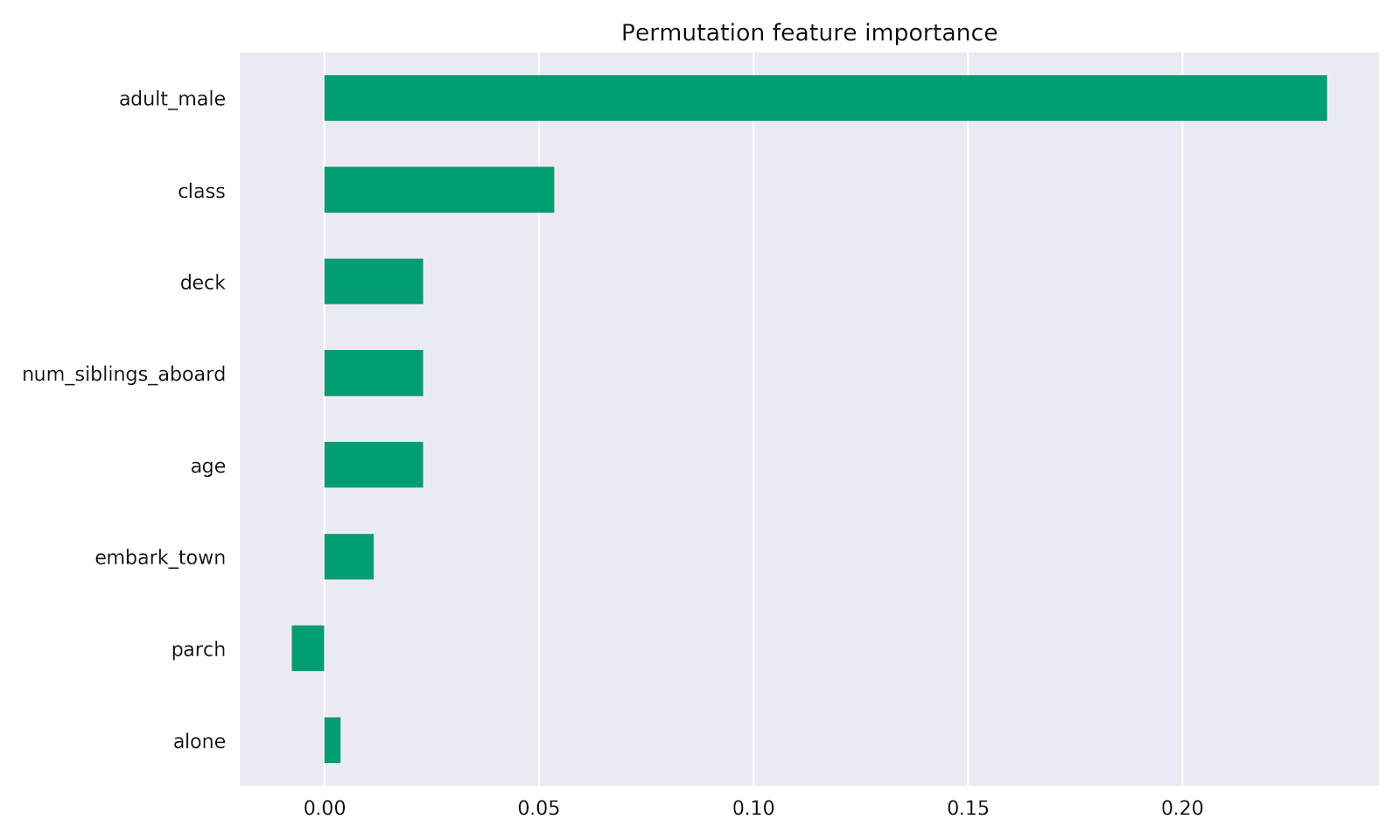How to train Boosted Trees models in TensorFlow
March 05, 2019

## And how to interpret them both locally and globally

Posted by Chris Rawles, Natalia Ponomareva, and Zhenyu Tan

``````## TL;DR:
# Train model.
est = tf.estimator.BoostedTreesClassifier(feature_columns, n_batches_per_layer)
est.train(train_input_fn)
# Per instance model interpretability:
pred_dict = est.experimental_predict_with_explanations(pred_input_fn)
# Global gain-based feature importances:
importances = est.experimental_feature_importances()``````
Tree ensemble methods such as gradient boosted decision trees and random forests are among the most popular and effective machine learning tools available when working with structured data. Tree ensemble methods are fast to train, work well without a lot of tuning, and do not require large datasets to train on.

In TensorFlow, gradient boosted trees are available using the `tf.estimator` API, which also supports deep neural networks, wide-and-deep models, and more. For boosted trees, regression with pre-defined mean squared error loss (`BoostedTreesRegressor`) and classification with cross entropy loss (`BoostedTreesClassifier`) are supported. Users can also choose to use any twice differentiable custom loss (by providing it to `BoostedTreesEstimator`).

In this post we will show how to train a Boosted Tree model in TensorFlow, then we’ll demonstrate how to interpret the trained model with feature importance and also how to interpret a model’s predictions for individual examples. All of the following code is TensorFlow 2.0 ready (premade estimators are fully supported in TensorFlow 2.0). All of the code in this post is available in the TensorFlow docs here and here.Visualizing the prediction surface of a Boosted Trees model. Gradient boosted trees is an ensemble technique that combines the predictions from several (think 10s, 100s or even 1000s) tree models. Increasing the number of trees will generally improve the quality of fit. Try the full example here.

### Training a Boosted Trees Model in TensorFlow

The Boosted Trees estimator supports large datasets that don’t fit in workers’ memory and it also provides distributed training. However, for demonstration purposes, let’s train a Boosted Trees model on a small dataset: the titanic dataset. The goal of this (rather morbid) dataset is to predict the probability that a passenger survived the titanic crash using passenger characteristics such as age, gender, class, etc.

First let’s import the necessary packages and load our dataset.
``````import numpy as np
import pandas as pd
import tensorflow as tf
tf.enable_eager_execution()

y_train = dftrain.pop('survived')
y_eval = dfeval.pop('survived')``````
Next, let’s define `feature_column`s to use with our `estimator` model. Feature columns work with all TensorFlow estimators and their purpose is to define the features used for modeling. Additionally they provide some feature engineering capabilities like one-hot-encoding, normalization, and bucketization. Below the fields in `CATEGORICAL_COLUMNS` are transformed from categorical columns to one-hot-encoded columns (indicator column):
``````fc = tf.feature_column
CATEGORICAL_COLUMNS = ['sex', 'n_siblings_spouses', 'parch', 'class', 'deck',
'embark_town', 'alone']
NUMERIC_COLUMNS = ['age', 'fare']

def one_hot_cat_column(feature_name, vocab):
return tf.feature_column.indicator_column(
tf.feature_column.categorical_column_with_vocabulary_list(feature_name,
vocab))
feature_columns = []
for feature_name in CATEGORICAL_COLUMNS:
# Need to one-hot encode categorical features.
vocabulary = dftrain[feature_name].unique()
feature_columns.append(one_hot_cat_column(feature_name, vocabulary))

for feature_name in NUMERIC_COLUMNS:
feature_columns.append(tf.feature_column.numeric_column(feature_name,
dtype=tf.float32))``````
You can view the transformation that a feature column produces. For example, here is the output when using the `indicator_column` on a single example:
``````example = dict(dftrain.head(1))
class_fc = tf.feature_column.indicator_column(tf.feature_column.categorical_column_with_vocabulary_list('class', ('First', 'Second', 'Third')))
print('Feature value: "{}"'.format(example['class'].iloc))
print('One-hot encoded: ', tf.keras.layers.DenseFeatures([class_fc])(example).numpy())

# Feature value: "Third"
# One-hot encoded:  [[0. 0. 1.]]``````
Next you need to create the input functions. These will specify how data will be read into our model for both training and inference. You will use the `from_tensor_slices` method in the tf.data API to read in data directly from Pandas. This is suitable for smaller, in-memory datasets. For larger datasets, the tf.data API supports a variety of file formats (including csv) so that you can process datasets that do not fit in memory.
``````# Use entire batch since this is such a small dataset.
NUM_EXAMPLES = len(y_train)

def make_input_fn(X, y, n_epochs=None, shuffle=True):
def input_fn():
dataset = tf.data.Dataset.from_tensor_slices((dict(X), y))
if shuffle:
dataset = dataset.shuffle(NUM_EXAMPLES)
# For training, cycle thru dataset as many times as need (n_epochs=None).
dataset = dataset.repeat(n_epochs)
# In memory training doesn't use batching.
dataset = dataset.batch(NUM_EXAMPLES)
return dataset
return input_fn

# Training and evaluation input functions.
train_input_fn = make_input_fn(dftrain, y_train)
eval_input_fn = make_input_fn(dfeval, y_eval, shuffle=False, n_epochs=1)``````
Let’s first train a logistic regression model to get a benchmark:
``````linear_est = tf.estimator.LinearClassifier(feature_columns)

# Train model.
linear_est.train(train_input_fn, max_steps=100)

# Evaluation.
result = linear_est.evaluate(eval_input_fn)``````
Then training a Boosted Trees model involves the same process as above:
``````# Since data fits into memory, use entire dataset per layer. It will be faster.
# Above one batch is defined as the entire dataset.
n_batches = 1
est = tf.estimator.BoostedTreesClassifier(feature_columns,
n_batches_per_layer=n_batches)

# The model will stop training once the specified number of trees is built, not
# based on the number of steps.
est.train(train_input_fn, max_steps=100)

# Eval.
result = est.evaluate(eval_input_fn)``````

### Model Understanding

For many end users the “why” and “how” are often as important as the prediction. For example, recent European Union regulation highlights users’ “right to explanation”, which dictates that users should be able to obtain an explanation for corporations’ decisions that significantly affect users (source). Additionally, the US Fair Credit Reporting Act requires that agencies disclose “all of the key factors that adversely affected the credit score of the consumer in the model used, the total number of which shall not exceed four” (source).

Model explainability can also help machine learning (ML) practitioners detect bias during the model development stage. Such insight helps ML practitioners better debug and understand their models.

There are generally two levels of model interpretability: local interpretability and global interpretability. Local interpretability refers to understanding a model’s predictions at the individual example level, while global interpretability refers to understanding the model as a whole.

Interpretability techniques are often specific to model types (e.g., tree methods, neural networks, etc.) and utilize the learned parameters. For example, gain-based feature importance is specific to tree methods, while the Integrated Gradients technique utilizes gradients in a neural network.

In contrast, there are also model-agnostic methods such as LIME and shap. LIME operates by building training a local surrogate model to approximate the predictions of the underlying black box model. The shap method connects game theory with local explanations by attributing to each feature the change in the expected model prediction when conditioning on that feature.

### Understanding individual predictions: Directional feature contributions

We have implemented the local feature contribution method outlined by Palczewska et al and by Saabas in Interpreting Random Forests. This method is also available in the treeinterpreter package for scikit-learn.

In short, the technique allows one to understand how a model makes a prediction for an individual instance by analyzing how the prediction changes when a split is added. Starting with the initial prediction (often referred to as the bias and is typically defined as the mean of the training labels), the technique traverses the prediction path, computing the change in the prediction after splitting on a feature. For each split, the change in prediction is attributed to the feature used for the split. Across all splits and all trees, these attributions are summed to indicate the total contributions of each feature.

The method returns a numeric value associated with each feature. We refer to these values as directional feature contributions (DFCs), to distinguish them from other ways of evaluating the impact of features, such as feature importance, which usually refer to global feature importance. DFCs allow for examination of individual examples and provide insight into why a model made a prediction for a particular example. Using this technique, you can create visualizations like this:DFCs for an instance in the titanic dataset. Informally this can be interpreted as “`adult_male` being True “contributes” about -0.11 to the final probability, while `Deck` being B contributes to about +0.08 to the final probability, and so on.”
A nice property of DFCs is that the sum of the contributions from each feature will sum up to the actual prediction. For example, if there are five features in the model and for a given instance the DFCs are

`{sex_female: 0.2, age: 0.05, fare= -0.02, num_siblings_aboard=-0.1, fare: 0.09}`

the predicted probability would be the sum of these values: 0.22.

We can also aggregate DFCs across the dataset to gain insight into the entire model for global interpretation:Mean absolute values of top DFCs across the entire evaluation dataset.`fare` value vs contributions with a LOWESS fit. Contributions across examples provide more granular information than a single feature importance metric. In general, a higher fare features results in the model pushing predictions closer to 1.0 (increasing chance of survival).

### How to: Directional feature contributions in TensorFlow

All of the code below is available in the Boosted Trees model understanding notebook.

First you need to train a Boosted Trees estimator using the tf.estimator API as described above.
``````params = {
'n_trees': 50,
'max_depth': 3,
'n_batches_per_layer': 1,
# You must enable center_bias = True to get DFCs. This will force the model to
# make an initial prediction before using any features (e.g. use the mean of
# the training labels for regression or log odds for classification when
# using cross entropy loss).
'center_bias': True
}
est = tf.estimator.BoostedTreesClassifier(feature_columns, **params)
# Train model.
est.train(train_input_fn, max_steps=100)
# Evaluation.
results = est.evaluate(eval_input_fn)``````
After training our model we can then retrieve model explanations using `est.experimental_predict_with_explanations`. (Note: The method is named experimental as we may modify the API before dropping the experimental prefix.)

Using pandas, you can easily visualize the DFCs:
``````# Make predictions.
pred_dicts = list(est.experimental_predict_with_explanations(eval_input_fn))
df_dfc = pd.DataFrame([pred['dfc'] for pred in pred_dicts])
# Plot results.
ID = 182
example = df_dfc.iloc[ID]  # Choose ith example from evaluation set.
TOP_N = 8  # View top 8 features.
sorted_ix = example.abs().sort_values()[-TOP_N:].index
ax = example[sorted_ix].plot(kind='barh')``````
In our Colab we have included example that adds the contributions distributions to understand how the DFCs for a particular instance compare to the rest of the evaluation set:Contributions for an individual example in red. The shaded blue area shows the distributions of contributions for features across the entire validation set.
We also note there are additional third-party model agnostic interpretability methods that work with TensorFlow such as LIME and shap. See the additional resources below for more links.

### Model-level interpretability: Gain-based and permutation feature importances

There are different ways to achieve model-level understanding (i.e., global interpretability) for Boosted Tree models. Earlier we showed that you can aggregate DFCs across the dataset for global interpretability. This also works by aggregating other local interpretation values such as those produced from LIME or shap (mentioned above).

Two other techniques we discuss below are Gain-based feature importances and permutation feature importance. Gain-based feature importances measure the loss change when splitting on a particular feature, while permutation feature importances are computed by evaluating model performance on the evaluation set by shuffling each feature one-by-one and attributing the loss in model performance to the shuffled feature. Permutation feature importance has the benefit of being model agnostic, however both methods can be unreliable in situations where potential predictor variables vary in their scale of measurement or their number of categories (source).

In the Boosted Trees estimators in TensorFlow, gain-based feature importances are retrieved using `est.experimental_feature_importances`. Here’s a full example with plotting:
``````# Get importances
importances = est.experimental_feature_importances(normalize=True)
df_imp = pd.Series(importances)

# Visualize importances.
N = 8
ax = (df_imp.iloc[0:N][::-1]
.plot(kind='barh'))``````Permutation feature importances can be computed as follows:
``````def permutation_importances(est, X_eval, y_eval, metric, features):
"""Column by column, shuffle values and observe effect on eval set.
source: http://explained.ai/rf-importance/index.html
A similar approach can be done during training. See "Drop-column importance"
in the above article."""
def accuracy_metric(est, X, y):
"""TensorFlow estimator accuracy."""
eval_input_fn = make_input_fn(X,
y=y,
shuffle=False,
n_epochs=1)
return est.evaluate(input_fn=eval_input_fn)['accuracy']

baseline = metric(est, X_eval, y_eval)
imp = []
for col in features:
save = X_eval[col].copy()
X_eval[col] = np.random.permutation(X_eval[col])
m = metric(est, X_eval, y_eval)
X_eval[col] = save
imp.append(baseline - m)
return np.array(imp)

importances = permutation_importances(est, dfeval, y_eval, accuracy_metric,
dftrain.columns)``````### Correlated variables and other considerations

Many model interpretation tools will provide a distorted view of feature impacts when the two or more features are correlated. For example, if you train an ensemble tree model containing two very correlated features, the gain-based feature importance of both features will be less compared to exclusively including either feature.

In the titanic dataset, let’s say we accidentally encoded a passenger’s class twice — in the form of two variables class and pclass. After encoding these categorical features using one-hot encoding and training the model, it’s observed that a passenger being in third class has prediction power — we can see this twice.After we drop one of the features (pclass) and re-examine feature importances, the significance of a passenger being in the third class approximately doubles.In this case the two features are perfectly correlated, however the same phenomenon occurs even with partially correlated features, just to a lesser extent.

Thus, for the techniques we’ve discussed above, it’s advisable to remove heavily correlated features. Not only will this aid interpretability, it will also result in faster model training. Plus maintaining fewer features is easier than maintaining a large number of features.

Finally, we note that Strobl et al. introduced another technique called conditional variable importance, utilizing feature permutation, that can help provide a more realistic estimate of feature impacts in the presence of correlated variables. Check out the paper for more details.

### Conclusion

Gradient boosted decision trees are available in TensorFlow using the `tf.estimator` API, which allows users to quickly experiment with different machine learning modes. For gradient boosted decision trees, local model interpretability (per-instance interpretability using the method outlined by Palczewska et al and by Saabas (Interpreting Random Forests) via `experimental_predict_with_explanations`) and global level interpretability (gain-based and permutation feature importances) are available in TensorFlow. These methods can help practitioners better understand their models.

The release of TensorFlow Boosted Trees has been possible thanks to a lot of people including, but not limited to Soroush Radpour, Younghee Kwon, Mustafa Ispir, Salem Haykal, and Yan Facai.